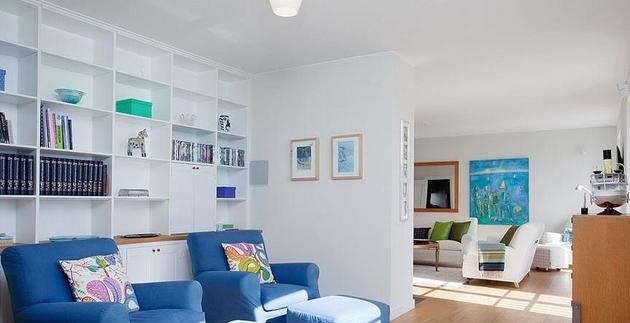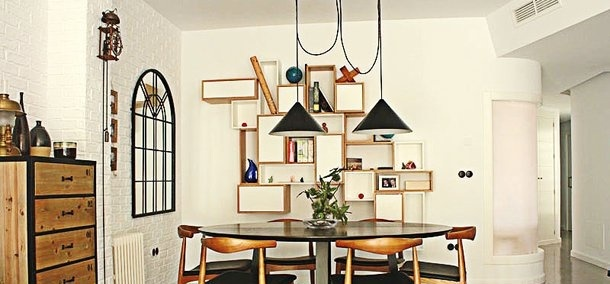|

# 旧房翻新刷墙预算 旧房翻新刷墙流程旧房翻新刷墙预算 旧房翻新刷墙流程

旧房翻新刷墙预算

1、旧房子翻新刷墙需要多少钱要根据自己使用的材料了，如果用的质量比较的好的油漆，那么价格也就会非常的高，有的一瓶就会是500——600元，这样的价格是非常贵的，当然贵也有贵的理由。质量好，价格肯定就会非常的高，小编觉得翻新旧的房子如果是自己住或者是让孩子结婚的，那么就应该选择质量比较好的油漆了，因为环保是非常重要。

2、旧房子翻新如果是租出去的，这个时候可以选择价格比较低的油漆，因为这个时候就是不想要旧房子看起来那么旧，所以翻新一下看起来会非常的好，并且租金也能上升的比较的快，当然这个时候粉刷的时候就可以选择每平方几十块钱的，这样刷出来的效果也会是非常好的。所以这就是小编给大家说的，粉刷的时候要看是用到什么地方的，然后对症下药就可以了。

旧房翻新刷墙流程

1、铲除表面油漆

与局部翻新一样，整体翻新同样需要铲掉表面涂层，注意必须把旧的腻子全部铲掉，铲到水泥层；如果墙面出现严重空鼓、粉化现象，建议铲到砖层，虽然花费的功夫较多，但能确保翻新后的墙面，既耐用又美观。为了使铲平时更方面，首先应该先将墙面沾水，润湿原有墙面，这样铲的时候省力。接着在已经被水浸过的部分铲墙皮，直到露出水泥砂浆墙面。

2、基层处理

铲完墙皮后要批刮腻子，选用颗粒细度较高和质地较硬的腻子为佳，在腻子里添加一定的白乳胶，可以提高腻子的硬度；然后检查裂缝，一般情况下，裂缝可用牛皮纸带和白乳胶贴住裂缝；接着在墙面涂刷界面剂，刷界面剂一定要都刷到，而且要刷匀；接着需要对墙面找平，一般用石膏对表面进行修补并用砂纸打磨，使墙面水平度误差为3mm以内，最后对墙面进行贴布。打磨时尽量用较细的砂纸，如果砂纸太粗的话会留下很深的砂痕，刷漆是覆盖不掉的。此外，轻体墙和保温墙等非承重墙都是需要贴布的，尽量选择质地好一点的墙布和白乳胶，如果有条件的话可以用网格布，相对防裂效果会更好一点。

3、防潮处理

墙面发霉的罪魁祸首就是受潮，所以在潮湿气候的地方一定要做好墙面的防潮处理，尤其是对于卫生间、厨房间的墙面做防潮处理。做防潮时要注意与防水区分开来，防潮必须墙面的水泥基面上做，不然是没有效果的，而且防潮需要在防水前做。这样就可以防止防水层的脱落，起泡。防潮需要选用专业的防潮材料，而并非防水材料。

4、重新刷漆

先用滚刷将大面积的部分涂刷完成，顺序为先吊顶、线条，后墙面。然后再用笔刷进行修补，特别是墙壁与角线、木柜、隔板等的接缝位置和墙壁的转角处。墙面上漆至少要上两遍，第一遍涂料可加5—10%的水，方便墙壁吸收，第二遍面漆可不加水，与第一遍涂层有一定的间隔时间。墙漆干透后，用砂纸对墙面进行打磨，以减少墙面刷痕。必须准备粗细两种滚筒，尽量选择好一点的工具，滚筒的毛不要太短，但一定要细，这样刷出来的漆膜才会手感细腻。此外，刷大面积时，图层相接的地方不能过厚也不能过薄，不然会因为薄厚不均造成反光不一致，视觉上就会有差异。

旧房翻新刷墙注意事项

注意事项1：墙漆满“五岁”必须铲除

对于购买的二手房墙面，如果只装修了一两年的房间，耐水腻子可以不必完全铲除，用钢刷和砂纸打磨墙面后即可重新涂刷乳胶漆。但是需要注意的是，五年以上的耐水腻子由于材料老化，在二次装修时必须铲除至墙底，否则会影响今后墙面乳胶漆的施工质量和装修后的长期使用效果。

注意事项2：墙面修补要全面

对于已上漆墙面的修补，一般来说很难达到理想效果。所以业主在对二手房墙面翻新时一定要修补全面，对于已经伤其墙面的修补最好是整块墙面或是尽可能地选择较大面积进行修补，这样不断可以尽量避免产生色差，修补后的质量也更好。

注意事项3：刷漆后需注意通风

此外，翻新后的二手房也要注意通风，尤其是对于老房子，其通风条件较差，稀释剂中的二甲苯成分会与乳胶漆中的成分发生化学反应，导致墙面泛黄。所以要加强通风，实在不行，得放置几台电风扇以加强通风效果。

注意事项4：拆改墙体要保证安全

对于二手房的装修格局，很可能不适合我们的意愿而对其进行拆改，合理的拆改是美化和调。但是要注意保证安全。依据相关法规，“非承重墙体是可以考虑拆改的。”同时，在拆除墙体时，要事先断电，避免发生事故。原有电源线路和弱电线路要保留的，最好事先做好记号和记录，最好不要切断视频线和宽带网路线，以防止装修后信号不通。某些和厨房、卫生间相邻的墙体最好不要拆除，拆除后会破坏防水层的完整性，影响今后居住安全。`声明：本文由入驻焦点开放平台的作者撰写，除焦点官方账号外，观点仅代表作者本人，不代表焦点立场错误信息举报电话： 400-099-0099，邮箱：jubao@vip.sohu.com，或点此进行意见反馈，或点此进行举报投诉。`A B C D E F G H J K L M N P Q R S T W X Y Z
A - B - C - D - E
• A
• 鞍山
• 安庆
• 安阳
• 安顺
• 安康
• 澳门
• B
• 北京
• 保定
• 包头
• 巴彦淖尔
• 本溪
• 蚌埠
• 亳州
• 滨州
• 北海
• 百色
• 巴中
• 毕节
• 保山
• 宝鸡
• 白银
• 巴州
• C
• 承德
• 沧州
• 长治
• 赤峰
• 朝阳
• 长春
• 常州
• 滁州
• 池州
• 长沙
• 常德
• 郴州
• 潮州
• 崇左
• 重庆
• 成都
• 楚雄
• 昌都
• 慈溪
• 常熟
• D
• 大同
• 大连
• 丹东
• 大庆
• 东营
• 德州
• 东莞
• 德阳
• 达州
• 大理
• 德宏
• 定西
• 儋州
• 东平
• E
• 鄂尔多斯
• 鄂州
• 恩施
F - G - H - I - J
• F
• 抚顺
• 阜新
• 阜阳
• 福州
• 抚州
• 佛山
• 防城港
• G
• 赣州
• 广州
• 桂林
• 贵港
• 广元
• 广安
• 贵阳
• 固原
• H
• 邯郸
• 衡水
• 呼和浩特
• 呼伦贝尔
• 葫芦岛
• 哈尔滨
• 黑河
• 淮安
• 杭州
• 湖州
• 合肥
• 淮南
• 淮北
• 黄山
• 菏泽
• 鹤壁
• 黄石
• 黄冈
• 衡阳
• 怀化
• 惠州
• 河源
• 贺州
• 河池
• 海口
• 红河
• 汉中
• 海东
• I
• J
• 晋中
• 锦州
• 吉林
• 鸡西
• 佳木斯
• 嘉兴
• 金华
• 景德镇
• 九江
• 吉安
• 济南
• 济宁
• 焦作
• 荆门
• 荆州
• 江门
• 揭阳
• 金昌
• 酒泉
• 嘉峪关
K - L - M - N - P
• K
• 开封
• 昆明
• 昆山
• L
• 廊坊
• 临汾
• 辽阳
• 连云港
• 丽水
• 六安
• 龙岩
• 莱芜
• 临沂
• 聊城
• 洛阳
• 漯河
• 娄底
• 柳州
• 来宾
• 泸州
• 乐山
• 六盘水
• 丽江
• 临沧
• 拉萨
• 林芝
• 兰州
• 陇南
• M
• 牡丹江
• 马鞍山
• 茂名
• 梅州
• 绵阳
• 眉山
• N
• 南京
• 南通
• 宁波
• 南平
• 宁德
• 南昌
• 南阳
• 南宁
• 内江
• 南充
• P
• 盘锦
• 莆田
• 平顶山
• 濮阳
• 攀枝花
• 普洱
• 平凉
Q - R - S - T - W
• Q
• 秦皇岛
• 齐齐哈尔
• 衢州
• 泉州
• 青岛
• 清远
• 钦州
• 黔南
• 曲靖
• 庆阳
• R
• 日照
• 日喀则
• S
• 石家庄
• 沈阳
• 双鸭山
• 绥化
• 上海
• 苏州
• 宿迁
• 绍兴
• 宿州
• 三明
• 上饶
• 三门峡
• 商丘
• 十堰
• 随州
• 邵阳
• 韶关
• 深圳
• 汕头
• 汕尾
• 三亚
• 三沙
• 遂宁
• 山南
• 商洛
• 石嘴山
• T
• 天津
• 唐山
• 太原
• 通辽
• 铁岭
• 泰州
• 台州
• 铜陵
• 泰安
• 铜仁
• 铜川
• 天水
• 天门
• W
• 乌海
• 乌兰察布
• 无锡
• 温州
• 芜湖
• 潍坊
• 威海
• 武汉
• 梧州
• 渭南
• 武威
• 吴忠
• 乌鲁木齐
X - Y - Z
• X
• 邢台
• 徐州
• 宣城
• 厦门
• 新乡
• 许昌
• 信阳
• 襄阳
• 孝感
• 咸宁
• 湘潭
• 湘西
• 西双版纳
• 西安
• 咸阳
• 西宁
• 仙桃
• 西昌
• Y
• 运城
• 营口
• 盐城
• 扬州
• 鹰潭
• 宜春
• 烟台
• 宜昌
• 岳阳
• 益阳
• 永州
• 阳江
• 云浮
• 玉林
• 宜宾
• 雅安
• 玉溪
• 延安
• 榆林
• 银川
• Z
• 张家口
• 镇江
• 舟山
• 漳州
• 淄博
• 枣庄
• 郑州
• 周口
• 驻马店
• 株洲
• 张家界
• 珠海
• 湛江
• 肇庆
• 中山
• 自贡
• 资阳
• 遵义
• 昭通
• 张掖
• 中卫

1室1厅1厨1卫1阳台

1
2
3
4
5

0
1
2

1

1

0
1
2
3报名成功，资料已提交审核A B C D E F G H J K L M N P Q R S T W X Y Z
A - B - C - D - E
• A
• 鞍山
• 安庆
• 安阳
• 安顺
• 安康
• 澳门
• B
• 北京
• 保定
• 包头
• 巴彦淖尔
• 本溪
• 蚌埠
• 亳州
• 滨州
• 北海
• 百色
• 巴中
• 毕节
• 保山
• 宝鸡
• 白银
• 巴州
• C
• 承德
• 沧州
• 长治
• 赤峰
• 朝阳
• 长春
• 常州
• 滁州
• 池州
• 长沙
• 常德
• 郴州
• 潮州
• 崇左
• 重庆
• 成都
• 楚雄
• 昌都
• 慈溪
• 常熟
• D
• 大同
• 大连
• 丹东
• 大庆
• 东营
• 德州
• 东莞
• 德阳
• 达州
• 大理
• 德宏
• 定西
• 儋州
• 东平
• E
• 鄂尔多斯
• 鄂州
• 恩施
F - G - H - I - J
• F
• 抚顺
• 阜新
• 阜阳
• 福州
• 抚州
• 佛山
• 防城港
• G
• 赣州
• 广州
• 桂林
• 贵港
• 广元
• 广安
• 贵阳
• 固原
• H
• 邯郸
• 衡水
• 呼和浩特
• 呼伦贝尔
• 葫芦岛
• 哈尔滨
• 黑河
• 淮安
• 杭州
• 湖州
• 合肥
• 淮南
• 淮北
• 黄山
• 菏泽
• 鹤壁
• 黄石
• 黄冈
• 衡阳
• 怀化
• 惠州
• 河源
• 贺州
• 河池
• 海口
• 红河
• 汉中
• 海东
• I
• J
• 晋中
• 锦州
• 吉林
• 鸡西
• 佳木斯
• 嘉兴
• 金华
• 景德镇
• 九江
• 吉安
• 济南
• 济宁
• 焦作
• 荆门
• 荆州
• 江门
• 揭阳
• 金昌
• 酒泉
• 嘉峪关
K - L - M - N - P
• K
• 开封
• 昆明
• 昆山
• L
• 廊坊
• 临汾
• 辽阳
• 连云港
• 丽水
• 六安
• 龙岩
• 莱芜
• 临沂
• 聊城
• 洛阳
• 漯河
• 娄底
• 柳州
• 来宾
• 泸州
• 乐山
• 六盘水
• 丽江
• 临沧
• 拉萨
• 林芝
• 兰州
• 陇南
• M
• 牡丹江
• 马鞍山
• 茂名
• 梅州
• 绵阳
• 眉山
• N
• 南京
• 南通
• 宁波
• 南平
• 宁德
• 南昌
• 南阳
• 南宁
• 内江
• 南充
• P
• 盘锦
• 莆田
• 平顶山
• 濮阳
• 攀枝花
• 普洱
• 平凉
Q - R - S - T - W
• Q
• 秦皇岛
• 齐齐哈尔
• 衢州
• 泉州
• 青岛
• 清远
• 钦州
• 黔南
• 曲靖
• 庆阳
• R
• 日照
• 日喀则
• S
• 石家庄
• 沈阳
• 双鸭山
• 绥化
• 上海
• 苏州
• 宿迁
• 绍兴
• 宿州
• 三明
• 上饶
• 三门峡
• 商丘
• 十堰
• 随州
• 邵阳
• 韶关
• 深圳
• 汕头
• 汕尾
• 三亚
• 三沙
• 遂宁
• 山南
• 商洛
• 石嘴山
• T
• 天津
• 唐山
• 太原
• 通辽
• 铁岭
• 泰州
• 台州
• 铜陵
• 泰安
• 铜仁
• 铜川
• 天水
• 天门
• W
• 乌海
• 乌兰察布
• 无锡
• 温州
• 芜湖
• 潍坊
• 威海
• 武汉
• 梧州
• 渭南
• 武威
• 吴忠
• 乌鲁木齐
X - Y - Z
• X
• 邢台
• 徐州
• 宣城
• 厦门
• 新乡
• 许昌
• 信阳
• 襄阳
• 孝感
• 咸宁
• 湘潭
• 湘西
• 西双版纳
• 西安
• 咸阳
• 西宁
• 仙桃
• 西昌
• Y
• 运城
• 营口
• 盐城
• 扬州
• 鹰潭
• 宜春
• 烟台
• 宜昌
• 岳阳
• 益阳
• 永州
• 阳江
• 云浮
• 玉林
• 宜宾
• 雅安
• 玉溪
• 延安
• 榆林
• 银川
• Z
• 张家口
• 镇江
• 舟山
• 漳州
• 淄博
• 枣庄
• 郑州
• 周口
• 驻马店
• 株洲
• 张家界
• 珠海
• 湛江
• 肇庆
• 中山
• 自贡
• 资阳
• 遵义
• 昭通
• 张掖
• 中卫• 手机• 分享
• 设计
免费设计
• 计算器
装修计算器
• 入驻
合作入驻
• 联系
联系我们
• 置顶
返回顶部